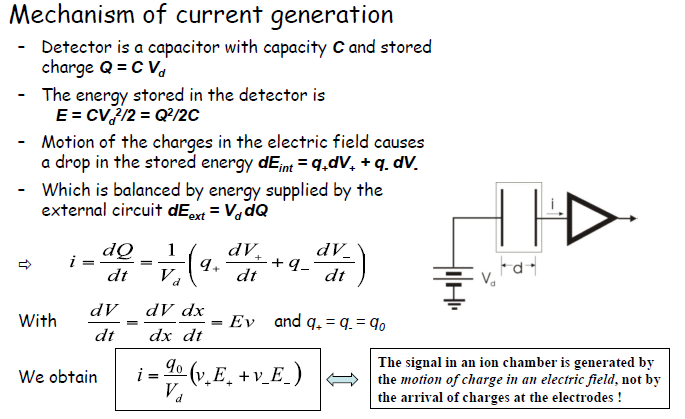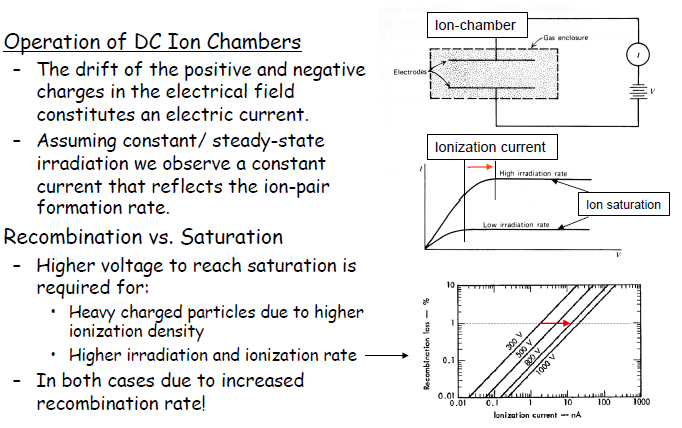## Pages

Showing posts with label gamma. Show all posts
Showing posts with label gamma. Show all posts

### What is Internal Conversion?

It is a process which enables an excited Nuclear state to come down to some lower state with out emission of a Gamma Photon. The energy ∆E involved in this Nuclear transition gets transferred directly to bound electron of atom. Such a electron gets knocked out of atom. Electrons like this are called conversion electrons and the process is called internal conversion.

It is interesting to note that wave mechanically, an atom electron spends part of its time inside a nucleus. This probability is highest for K-shell electrons which are closest to Nucleus. For such a case, Nucleus may de excite not by Ɣ- emission but by giving excitation energy ∆E directly to a K-shell electron.

Internal conversion is also possible for higher atomic levels L,M etc.

The kinetic energy of converted electron 'Ke' is Ke=∆E-Bₑ

Bₑ - atomic binding energy of electron

∆E = Ei-Ef ; Nuclear Excitation energy

Usually continuous 𝜷-spectra are super imposed by discrete lines due to conversion.

It was wrongly believed that internal conversion process is like Photoelectric effect; a Ɣ-photon emitted by a nucleus is absorbed by orbital electron which is emitted as in photoelectric effect.

The simplest situation which disproves this is a transition between two states having spin equal to zero.

A 0→0 transition (∆I=0) is forbidden for all multipole orders and so Ɣ-emission by nucleus is completely forbidden.

However 0→0 transition is readily found to proceed by internal conversion. The experiment was performed on 0.7MeV level of ⁷²Ge. This is a 0→0 transition and it was found that conversion electrons can be detected, but there is a complete absence of Ɣ- ray emission.

In 1932, Taylor and Mott suggested that transition probability 'λ' from a Nuclear state 'a' to a Nuclear state 'b' is sum of two terms

λ=λₑ+λᵧ

λₑ & λᵧ are partial decay constants for conversion electron emission  and for gamma emission respectively.

Ratio between two decay constants is called conversion coefficeint and is measured as ratio between total number of conversion electrons emitted  (N) and total no. of gamma rays (N) emitted in same transition over the same time.

Conversion Coeff(α) = Nₑ/Nᵧ=  λₑ/λᵧ

value of 'α' is found to depend on transition energy, multipole character of transition and atomic number Z.

### MECHANISM OF CURRENT GENERATION IN GAS FILLED IONIZATION CHAMBERScourtesy: Spring 2010 Radiation Detection & Measurements